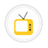you are here->home->Physical Sciences->Laser Optics Virtual Lab
Laser Optics Virtual Lab

This lab is thoroughly outfitted for experiments in introductory and advanced laser physics. It explains the study of optical properties for different material by adopting laser devices and handling basic aspects of interferometry.Michelson's Interferometer- Refractive index of glass plate Interferometers are basic optical tools used to precisely measure wavelength, distance, index of refraction, and temporal coherence of optical beams. Using Michelson Interferometer, one can to calculate the refractive index of a thin film.Newton's Rings-Refractive index of liquidRefractive index(index of refraction) is a dimensionless number which helps to determine the amount in which a ray bends when passing through a medium.Michelson's Interferometer- Wavelength of laser beam Using Michelson Interferometer, one can determine the wavelength of light from a monochromatic source.it can also be used to calculate the refractive index of a thin film.Laser beam divergence and spot sizeTo measure the Beam Spot size and Beam Divergence of a Laser beam using a detector in various ways. The beam profile is Gaussian. A Gaussian beam is a beam of electromagnetic radiation whose transverse electric field and intensity (irradiance) distributioNewton's Rings-Wavelength of lightNewton's rings is an interference pattern caused by the reflection of light between two surfaces - a spherical surface and an adjacent flat surface.Brewsters Angle determinationBrewster's angle (also known as the polarization angle) is an angle of incidence at which light with a particular polarization is perfectly transmitted through a surface, with no reflection.Numerical Aperture of Optical FiberOptical fibers are fine transparent glass or plastic fibers which propagate light using the phenomenon of total internal reflection from diametrically opposite walls. In this way light may be taken anywhere because fibers have enough flexibility. This makCopyright @ 2019 Under the NME ICT initiative of MHRDPowered by AmritaVirtual Lab Collaborative Platform [ Ver 00.13. ]